##Conic through four points and tangent to a line II

Here I discuss the problem of finding a conic passing through four points {A,B,C,D} and tangent to a line (t) passing through one of the points, D say. The case in which the line does not contain any of the four points is handled in FourPtsAndTangent.html .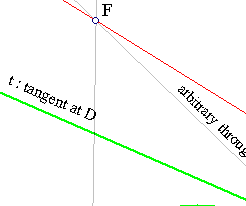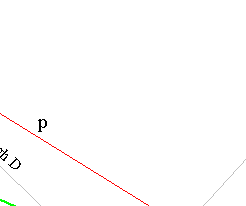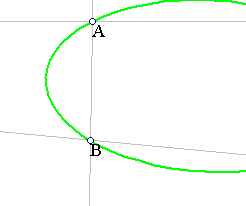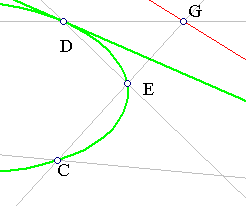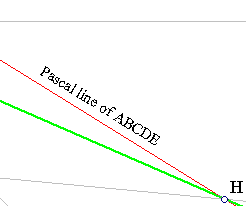The construction follows the recipe exposed in Pascal2.html . From the given data we determine first the intersection point H of (t) with BC. Then draw an arbitrary line DE through D intersecting AB at F. In order for E to be on the conic, the intersection point G of AD and CE must be collinear with F and H. Then the line p containing {F,G,H} will be the one whose existence is guaranteed from the theorem of Pascal.
Thus, G is determined on FH by intersecting it with AD and E is found as intersection of GC and FD. This reduces the problem to the one of finding a conic through the five points {A,B,C,D,E}.

CrossRatio0.html
DesarguesInvolution.html
FourPtsAndTangent.html
HomographicRelation.html
HomographicRelationExample.html
InvolutionBasic.html
InvolutionBasic2.html
Pascal.html
Pascal2.html

### References

Doerrie, Heinrich 100 Great Problems of Elementary Mathematics New York, Dover, 1965, p. 269.